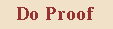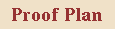# Proof Example 1: Remainder ProgramConsider this program: it computes a remainder

```
{ PRE: x >= 0 }
begin

q := 0

r := x

while r >= y do

r := r - y

q := q + 1

end

end
{ POST: 0 <= r < y  and  x = q * y + r }

```
Prove that the program is correct with respect to the given PRE and POST specifications...